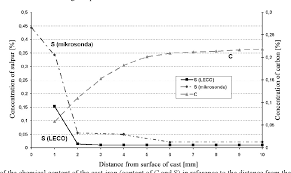## How to Calculate and Solve for Knudsen Diffusion of Moulding Sand | Mass TransferThe image above represents knudsen diffusion of moulding sand.

To compute for knudsen diffusion of moulding sand, three essential parameters are needed and these parameters are Pore Radius (r), Temperature (T) and Molecular Weight (M).

The formula for calculating knudsen diffusion of moulding sand:

Dk = 9700r√(T/M)

Where:

Dk = Knudsen Diffusion of Moulding Sand
T = Temperature
M = Molecular Weight

Let’s solve an example;
Find the knudsen diffusion of moulding sand when the pore radius is 24, the temperature is 18 and the molecular weight is 12.

This implies that;

r = Pore Radius = 24
T = Temperature = 18
M = Molecular Weight = 12

Dk = 9700r√(T/M)
Dk = 9700(24)√(18/12)
Dk = 9700(24)√(1.5)
Dk = 9700(24)(1.22)
Dk = 285120.6

Therefore, the knudsen diffusion of moulding sand is 285120.6 cm²/s.

Calculating the Pore Radius when the Knudsen Diffusion of Moulding Sand, the Temperature and the Molecular Weight is Given.

r = Dk / 9700 √(T / M)

Where:

Dk = Knudsen Diffusion of Moulding Sand
T = Temperature
M = Molecular Weight

Let’s solve an example;
Find the pore radius when the knudsen diffusion of moulding sand is 20, the temperature is 14 and the molecular weight is 10.

This implies that;

Dk = Knudsen Diffusion of Moulding Sand = 20
T = Temperature = 14
M = Molecular Weight = 10

r = Dk / 9700 √(T / M)
r = 20 / 9700 √(14 / 10)
r = 20 / 9700 √(1.4)
r = 20 / 11446
r = 0.00174

Therefore, the pore radius is 0.00174.